# GRE Quantitative Comparison Practice Problems

Here are ten GRE math practice questions to get you ready for Quantitative Comparison. All of these can and should be done without a calculator. Solutions will come at the end of this article.

1)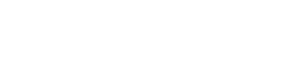2) The number n is an integer greater than 2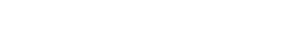3) The number x satisfies the restriction4)5) Set S consists of the elements {a, b, b, c} where 0 < a < b < c.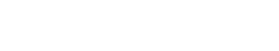6)7)8)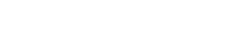9)10)## The GRE Quantitative Comparison questions

The QC questions are unique to the GRE: no other standardized test has anything quite like the QC questions.  Here are a few thoughts on QC strategy:

1) Compare, don’t calculate: Your job is to figure out which quantity is bigger, or whether they are equal.  You do have to compare the quantities.  You don’t have to perform a detailed calculation.  The GRE is not interested in your ability to perform detailed, time-consuming calculations.  The GRE is keenly interested in your ability to use logic and mathematical thinking to find shortcuts that radically simplify the comparisons in the QC.  If you find yourself getting sucked into a tedious, time-consuming calculation, probably you are doing something the long hard way.

2) Matching operations: With equations, you can always do the same thing to both sides of an equation.  Inequalities have a few more restrictions.  In a QC, the relationship between the two quantities could be an inequality, so we must restrict ourselves to what we can do to both sides of an inequality.  We can always add the same number to both quantities, or subtract the same number from both quantities: we can add or subtract anything, no limits.  With multiplication and division, we have to be guaranteed that the number is positive: we can multiply or divide both sides by any positive number.  We cannot multiple or divide by a variable unless we are guaranteed that the variable is always positive.

3) Picking numbers?  This strategy is not nearly as helpful on algebraic QCs as it is on algebraic multiple choice.   If you are running out of time, and need to guess, then picking numbers is very efficient: for example, if I pick one value for x, plug it in, and find, say, that Quantity A is bigger, then the answer has to be (A) or (D).  I have effectively eliminated both (B) & (C) as answers.  That would allow you to performed leveraged guessing in a very short amount of time.

If you are not running out of time, and have the ordinary time to devote to a QC question, then picking numbers, alone, will only be definitively helpful when the answer is (D).  You see, suppose I plug in one value of x and find, say, that Quantity A is bigger, and then I pick another value, and find, say, that the two quantities are equal.  As soon as two different choices lead to two different relationships of the quantities, then I know that (D) is the answer.  Remember, (D) is the answer about 25% of the time.  If I pick 3, or 5, different values, and each one makes Quantity A bigger, that’s no guarantee: I can’t rely on picking numbers alone to verify, beyond doubt, that (A) or (B) or (C) is the answer.  If I suspect that, I have to use logic or mathematical reasoning to verify.  You will see some examples of this in the solutions below.

## Summary

If the above paragraph gave you some flashes of insight, you may want to give the problems above another look before reading the solutions below.  Best of luck!

## Practice problem explanations

1) When both quantities are positive, as these are, we are allowed to square both quantities, because for positive numbers, squaring preserves the order of inequality.2) This one is relatively straightforward and lends itself well to picking numbers.

For n = 3: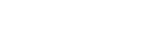So, for this choice, quantity B is bigger.  Now, try n = 4.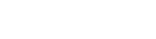For this choice, they are equal.  Two different numerical choices lead to two different relationships between the quantities.

3) On this one, picking numbers won’t get us very far.  Notice, first of all, that x could be positive or negative.  If x is positive, it must be greater than 1.  If x is negative, it must be less than – 1.  Right there, we have our answer.  If a number has an absolute value greater than 1, then squaring the number always increases the absolute value.

4) This is very tricky one.  When x = 0, the quadratic equals 5/12, which is positive.  For any positive number, whole or fraction we would simply be adding three positive numbers, so this is still positive, greater than zero.  Rather than pick more numbers, which could get complicated, let’s think about this.

If we were to graph this on the x-y plane, this would be a parabola.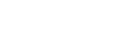The two “arms” point upward and definitely would be positive.  The question is whether the lowest point, the vertex, would be positive or negative.   Here are a couple advanced facts about parabolas that you could need if you are seeing some of the harder Quant questions on test day.  Fact #1: the vertex of any parabola lies on its line of symmetry.  Fact #2: the line of symmetry has the equation: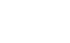where a is the quadratic coefficient, that is, the coefficient of x squared; and b is the linear coefficient, that is, the coefficient of x.  Here, a = 1, and b = 4/3, so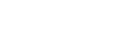Plug this into the equation:So, the vertex is just below zero, so the expression can be negative as well as positive.

5) Here, we will pick some numbers, but first, let’s think.  For symmetrical sets, the mean and the median are relatively close or even equal.  When an outlier on one side of the set of numbers lies far away from all the other numbers, this outlier pulls the mean away from the median.  The mean gets tugged in the direction of an outlier, whereas the median is impervious.

First, pick a = 1, b = 2, c = 3, a nice easy symmetrical set. S = {1, 2, 2, 3}.  For this set, mean = median = 2, so the two quantities are equal for these choices.

Now, change it so that one number is an outlier.  Pick a = 1, b = 2, and c = 500.  S = {1, 2, 2, 500}.  Notice that the median is still 2, the average of the two central 2’s.  In contrast, the mean now would be 505/4.  We don’t need to calculate that: it’s obviously larger than two!  The mean is now bigger.

Different choices of numbers produce different relationships of the quantities.

6) All three variables have to be positive, because they are lengths.  This means that (c + a) also must be positive, so we can multiply both quantities by this.  This will produce the Difference of Two Squares pattern in Quantity B.Of course, the Pythagorean Theorem tells us these quantities must be equal for any right triangle on the planet, so we know these two columns are always equal.

7) For this one, instead of picking numbers, let’s “solve” the two quantities, isolating x in one of the two quantities.Well, if x is greater than 2, then it could be bigger than two and one sixth, or it could be equal, or it could even be less: for example x = 2 + (1/100) is greater than two and less than two and one sixth.  Different choices of numbers produce different relationships of the quantities.

8) For this one, plugging numbers is not the best way to go.  Instead, it good to recognize the algebraic pattern, the Square of a Sum.  (For details, see this GMAT post.)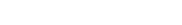Here,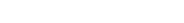This is very close to what we have in quantity A.  In fact, we could say: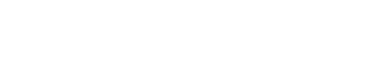Quantity A equals something squared (always zero or positive) plus 3, so this sum is always positive, always greater than zero.

9) It would be cumbersome and problematic to plug in numbers to this one.  Instead, notice the following about the prompt equation.  Subtract w from both sides.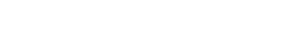Now, multiply both sides by that denominator, (m + w):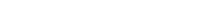On the right side, we have the Difference of Two Squares pattern.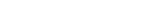Now, simply add w squared to both sides.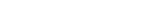Adding one to any number on the number line makes it bigger.  For any k, k < k + 1.  Thus: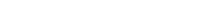Thus, m squared is always bigger than the sum of the squares in Quantity A.

10) Picking number is not the best approach for this.  This one is much more readily dispatched using algebraic simplification of the prompt equation.

First, subtract 1 from both sides of that starting equation, then divide both sides by 3 to simplify.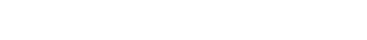Because this is an equation, we can multiply both side by any number. Multiply by the denominator (B + 3A).

4A = B + 3A

Subtract 3A from both sides.

A = B

Thus, the quantities have to be equal!

•